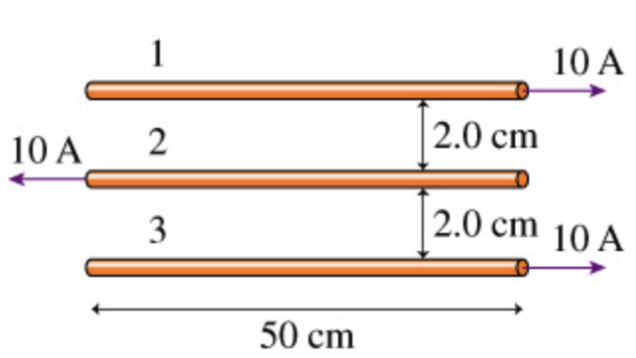In: Physics

# What is the magnitude of the net force on the first wire in (figure 1)?

Figure 1What is the magnitude of the net force on the first wire in (Figure 1)?

What is the magnitude of the net force on the second wire in (Figure 1)?

What is the magnitude of the net force on the third wire in (Figure 1)?

## Solutions

##### Expert Solution

From the diagram, you can see that wire 1 has an attracting force from wire 3 at 0.04 m distance and a repelling force from wire 2 at 0.02 m distance.

Expression of the force is

F = µ*I1*I2*L/(2πd)

When d = 0.02 m,

F = (4*pi*10^-7*10*10*0.50) / (2*pi*0.02) = 0.0005 N

And when d = 0.04 m,

F = (4*pi*10^-7*10*10*0.50) / (2*pi*0.04) = 0.00025 N

(a) So, the net force on wire 1 = 0.005 - 0.00025 = 0.00025 N repelling, or upward.

(b) Wire 2 has equal repelling forces from wires 1 and 3, so net force = 0.

(c) By symmetry, the net force on wire 3 is the same means, 0.00025 N, but downward direction.

## Related Solutions

##### In (Figure 1), charge q2 experiences no net electric force. What is q1?
In (Figure 1), charge q2 experiences no net electric force. What is q1?
##### What is the magnitude of the electric force on charge A in the figure?
Part A What is the magnitude of the electric force on charge A in the figure?F=______N Part BWhat is the direction of the electric force on charge A in the figure? Choose best answer. (a)to the left (b)to the right (c)the force is zero
##### What is the magnitude of the electric field at the dot in the figure? (Figure 1)
Part A What is the magnitude of the electric field at the dot in the figure? (Figure 1) Express your answer using two significant figures. E = _________V/m   Part B  What is the direction of the electric field at the dot in the figure? Choose the best answer. (a) the negative x-axis. (b) the positive x-axis. (c) 45 below -x-axis (d) 45 below +x-axis
##### The current in the wire shown in (Figure 1) is increasing
The current in the wire shown in (Figure 1) is increasing. Part A What is the direction of the induced current in loop A? clockwise counterclockwise No current is induced in this loop.   Part B What is the direction of the induced current in loop B? clockwise counterclockwise No current is induced in this loop.
##### Determine the magnitude and direction of the electric field at point 1 in the figure(Figure 1).
Figure 1 Part A Determine the magnitude and direction of the electric field at point 1 in the figure(Figure 1). E1→=(2500V/m,up) E1→=(7500V/m,up) E1→=(3750V/m,down) E1→=(2500V/m,down) Part B Determine the magnitude and direction of the electric field at point 2 in the figure. E2→=(2500V/m,up) E2→=(3750V/m,down) E2→=(7500V/m,down) E2→=(5000V/m,up)
##### What are the magnitude and direction of the current in the 20 Ω resistor in (Figure 1)?
(Figure 1) What are the magnitude and direction of the current in the 20 Ω resistor in (Figure 1)? Express your answer with the appropriate units. Enter positive value if the current is clockwise and negative value if the current is counterclockwise.
##### What is the equivalent capacitance for the circuit of the figure? (Figure 1)
Part A What is the equivalent capacitance for the circuit of the figure? (Figure 1)Express your answer to two significant figures and include the appropriate units. Part B How much charge flows through the battery as the capacitors are being charged? Express your answer to two significant figures and include the appropriate units.
##### What is the time constant for the discharge of the capacitors in the figure (Figure 1) ?
What is the time constant for the discharge of the capacitors in the figure (Figure 1)?
##### What is the magnetic field at the center of the loop in the figure? (figure 1)
Figure 1 What is the magnetic field at the center of the loop in the figure? (Figure 1)
##### What magnitude and sign of charge Q will make the force on charge q zero?
(Figure 1) shows four charges at the corners of a square of side L. What magnitude and sign of charge Q will make the force on charge q zero? Q =